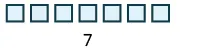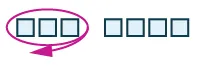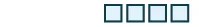Prealgebra

# 1.3Subtract Whole Numbers

Prealgebra1.3 Subtract Whole Numbers

### Learning Objectives

By the end of this section, you will be able to:
• Use subtraction notation
• Model subtraction of whole numbers
• Subtract whole numbers
• Translate word phrases to math notation
• Subtract whole numbers in applications

### Be Prepared 1.2

Before you get started, take this readiness quiz.

1. Model $3+43+4$ using base-ten blocks.
If you missed this problem, review Example 1.12.
2. Add: $324+586.324+586.$
If you missed this problem, review Example 1.20.

### Use Subtraction Notation

Suppose there are seven bananas in a bowl. Elana uses three of them to make a smoothie. How many bananas are left in the bowl? To answer the question, we subtract three from seven. When we subtract, we take one number away from another to find the difference. The notation we use to subtract $33$ from $77$ is

$7−37−3$

We read $7−37−3$ as seven minus three and the result is the difference of seven and three.

### Subtraction Notation

To describe subtraction, we can use symbols and words.

Operation Notation Expression Read as Result
Subtraction $−−$ $7−37−3$ seven minus three the difference of $77$ and $33$

### Example 1.26

Translate from math notation to words: $8−18−1$ $26−1426−14$.

### Try It 1.51

Translate from math notation to words:

1. $12−412−4$
2. $29−1129−11$

### Try It 1.52

Translate from math notation to words:

1. $11−211−2$
2. $29−1229−12$

### Model Subtraction of Whole Numbers

A model can help us visualize the process of subtraction much as it did with addition. Again, we will use $base-10base-10$ blocks. Remember a block represents 1 and a rod represents 10. Let’s start by modeling the subtraction expression we just considered, $7−3.7−3.$

 We start by modeling the first number, 7.Now take away the second number, 3. We'll circle 3 blocks to show that we are taking them away.Count the number of blocks remaining.There are 4 ones blocks left. We have shown that $7−3=47−3=4$.

### Manipulative Mathematics

Doing the Manipulative Mathematics activity Model Subtraction of Whole Numbers will help you develop a better understanding of subtracting whole numbers.

### Example 1.27

Model the subtraction: $8−2.8−2.$

### Try It 1.53

Model: $9−6.9−6.$

### Try It 1.54

Model: $6−1.6−1.$

### Example 1.28

Model the subtraction: $13−8.13−8.$

### Try It 1.55

Model the subtraction: $12−7.12−7.$

### Try It 1.56

Model the subtraction: $14−8.14−8.$

### Example 1.29

Model the subtraction: $43−26.43−26.$

### Try It 1.57

Model the subtraction: $42−27.42−27.$

### Try It 1.58

Model the subtraction: $45−29.45−29.$

### Subtract Whole Numbers

We know $7−3=47−3=4$ because $4+3=7.4+3=7.$ Knowing all the addition number facts will help with subtraction. Then we can check subtraction by adding. In the examples above, our subtractions can be checked by addition.

$7−3=4because4+3=713−8=5because5+8=1343−26=17because17+26=437−3=4because4+3=713−8=5because5+8=1343−26=17because17+26=43$

### Example 1.30

Subtract and then check by adding:

1. $9−79−7$
2. $8−3.8−3.$

### Try It 1.59

Subtract and then check by adding:

$7−07−0$

### Try It 1.60

Subtract and then check by adding:

$6−26−2$

To subtract numbers with more than one digit, it is usually easier to write the numbers vertically in columns just as we did for addition. Align the digits by place value, and then subtract each column starting with the ones and then working to the left.

### Example 1.31

Subtract and then check by adding: $89−61.89−61.$

### Try It 1.61

Subtract and then check by adding: $86−54.86−54.$

### Try It 1.62

Subtract and then check by adding: $99−74.99−74.$

When we modeled subtracting $2626$ from $43,43,$ we exchanged $11$ ten for $1010$ ones. When we do this without the model, we say we borrow $11$ from the tens place and add $1010$ to the ones place.

### How To

#### Find the difference of whole numbers.

1. Step 1. Write the numbers so each place value lines up vertically.
2. Step 2. Subtract the digits in each place value. Work from right to left starting with the ones place. If the digit on top is less than the digit below, borrow as needed.
3. Step 3. Continue subtracting each place value from right to left, borrowing if needed.
4. Step 4. Check by adding.

### Example 1.32

Subtract: $43−26.43−26.$

### Try It 1.63

Subtract and then check by adding: $93−58.93−58.$

### Try It 1.64

Subtract and then check by adding: $81−39.81−39.$

### Example 1.33

Subtract and then check by adding: $207−64.207−64.$

### Try It 1.65

Subtract and then check by adding: $439−52.439−52.$

### Try It 1.66

Subtract and then check by adding: $318−75.318−75.$

### Example 1.34

Subtract and then check by adding: $910−586.910−586.$

### Try It 1.67

Subtract and then check by adding: $832−376.832−376.$

### Try It 1.68

Subtract and then check by adding: $847−578.847−578.$

### Example 1.35

Subtract and then check by adding: $2,162−479.2,162−479.$

### Try It 1.69

Subtract and then check by adding: $4,585−697.4,585−697.$

### Try It 1.70

Subtract and then check by adding: $5,637−899.5,637−899.$

### Translate Word Phrases to Math Notation

As with addition, word phrases can tell us to operate on two numbers using subtraction. To translate from a word phrase to math notation, we look for key words that indicate subtraction. Some of the words that indicate subtraction are listed in Table 1.3.

Operation Word Phrase Example Expression
Subtraction minus $55$ minus $11$ $5−15−1$
difference the difference of $99$ and $44$ $9−49−4$
decreased by $77$ decreased by $33$ $7−37−3$
less than $55$ less than $88$ $8−58−5$
subtracted from $11$ subtracted from $66$ $6−16−1$
Table 1.3

### Example 1.36

Translate and then simplify:

1. the difference of $1313$ and $88$
2. subtract $2424$ from $4343$

### Try It 1.71

Translate and simplify:

1. the difference of $1414$ and $99$
2. subtract $2121$ from $3737$

### Try It 1.72

Translate and simplify:

1. $1111$ decreased by $66$
2. $1818$ less than $6767$

### Subtract Whole Numbers in Applications

To solve applications with subtraction, we will use the same plan that we used with addition. First, we need to determine what we are asked to find. Then we write a phrase that gives the information to find it. We translate the phrase into math notation and then simplify to get the answer. Finally, we write a sentence to answer the question, using the appropriate units.

### Example 1.37

The temperature in Chicago one morning was $7373$ degrees Fahrenheit. A cold front arrived and by noon the temperature was $2727$ degrees Fahrenheit. What was the difference between the temperature in the morning and the temperature at noon?

### Try It 1.73

The high temperature on $June1stJune1st$ in Boston was $7777$ degrees Fahrenheit, and the low temperature was $5858$ degrees Fahrenheit. What was the difference between the high and low temperatures?

### Try It 1.74

The weather forecast for June $22$ in St Louis predicts a high temperature of $9090$ degrees Fahrenheit and a low of $7373$ degrees Fahrenheit. What is the difference between the predicted high and low temperatures?

### Example 1.38

A washing machine is on sale for $399.399.$ Its regular price is $588.588.$ What is the difference between the regular price and the sale price?

### Try It 1.75

A television set is on sale for $499.499.$ Its regular price is $648.648.$ What is the difference between the regular price and the sale price?

### Try It 1.76

A patio set is on sale for $149.149.$ Its regular price is $285.285.$ What is the difference between the regular price and the sale price?

### Section 1.3 Exercises

#### Practice Makes Perfect

Use Subtraction Notation

In the following exercises, translate from math notation to words.

141.

$15 − 9 15 − 9$

142.

$18 − 16 18 − 16$

143.

$42 − 35 42 − 35$

144.

$83 − 64 83 − 64$

145.

$675 − 350 675 − 350$

146.

$790 − 525 790 − 525$

Model Subtraction of Whole Numbers

In the following exercises, model the subtraction.

147.

$5 − 2 5 − 2$

148.

$8 − 4 8 − 4$

149.

$6 − 3 6 − 3$

150.

$7 − 5 7 − 5$

151.

$18 − 5 18 − 5$

152.

$19 − 8 19 − 8$

153.

$17 − 8 17 − 8$

154.

$17 − 9 17 − 9$

155.

$35 − 13 35 − 13$

156.

$32 − 11 32 − 11$

157.

$61 − 47 61 − 47$

158.

$55 − 36 55 − 36$

Subtract Whole Numbers

In the following exercises, subtract and then check by adding.

159.

$9 − 4 9 − 4$

160.

$9 − 3 9 − 3$

161.

$8 − 0 8 − 0$

162.

$2 − 0 2 − 0$

163.

$38 − 16 38 − 16$

164.

$45 − 21 45 − 21$

165.

$85 − 52 85 − 52$

166.

$99 − 47 99 − 47$

167.

$493 − 370 493 − 370$

168.

$268 − 106 268 − 106$

169.

$5,946 − 4,625 5,946 − 4,625$

170.

$7,775 − 3,251 7,775 − 3,251$

171.

$75 − 47 75 − 47$

172.

$63 − 59 63 − 59$

173.

$461 − 239 461 − 239$

174.

$486 − 257 486 − 257$

175.

$525 − 179 525 − 179$

176.

$542 − 288 542 − 288$

177.

$6,318 − 2,799 6,318 − 2,799$

178.

$8,153 − 3,978 8,153 − 3,978$

179.

$2,150 − 964 2,150 − 964$

180.

$4,245 − 899 4,245 − 899$

181.

$43,650 − 8,982 43,650 − 8,982$

182.

$35,162 − 7,885 35,162 − 7,885$

Translate Word Phrases to Algebraic Expressions

In the following exercises, translate and simplify.

183.

The difference of $1010$ and $33$

184.

The difference of $1212$ and $88$

185.

The difference of $1515$ and $44$

186.

The difference of $1818$ and $77$

187.

Subtract $66$ from $99$

188.

Subtract $88$ from $99$

189.

Subtract $2828$ from $7575$

190.

Subtract $5959$ from $8181$

191.

$4545$ decreased by $2020$

192.

$3737$ decreased by $2424$

193.

$9292$ decreased by $6767$

194.

$7575$ decreased by $4949$

195.

$1212$ less than $1616$

196.

$1515$ less than $1919$

197.

$3838$ less than $6161$

198.

$4747$ less than $6262$

Mixed Practice

In the following exercises, simplify.

199.

$76 − 47 76 − 47$

200.

$91 − 53 91 − 53$

201.

$256 − 184 256 − 184$

202.

$305 − 262 305 − 262$

203.

$719 + 341 719 + 341$

204.

$647 + 528 647 + 528$

205.

$2,015 − 1,993 2,015 − 1,993$

206.

$2,020 − 1,984 2,020 − 1,984$

In the following exercises, translate and simplify.

207.

Seventy-five more than thirty-five

208.

Sixty more than ninety-three

209.

$1313$ less than $4141$

210.

$2828$ less than $3636$

211.

The difference of $100100$ and $7676$

212.

The difference of $1,0001,000$ and $945945$

Subtract Whole Numbers in Applications

In the following exercises, solve.

213.

Temperature The high temperature on June $22$ in Las Vegas was $8080$ degrees and the low temperature was $6363$ degrees. What was the difference between the high and low temperatures?

214.

Temperature The high temperature on June $11$ in Phoenix was $9797$ degrees and the low was $7373$ degrees. What was the difference between the high and low temperatures?

215.

Class size Olivia’s third grade class has $3535$ children. Last year, her second grade class had $2222$ children. What is the difference between the number of children in Olivia’s third grade class and her second grade class?

216.

Class size There are $8282$ students in the school band and $4646$ in the school orchestra. What is the difference between the number of students in the band and the orchestra?

217.

Shopping A mountain bike is on sale for $399.399.$ Its regular price is $650.650.$ What is the difference between the regular price and the sale price?

218.

Shopping A mattress set is on sale for $755.755.$ Its regular price is $1,600.1,600.$ What is the difference between the regular price and the sale price?

219.

Savings John wants to buy a laptop that costs $840.840.$ He has $685685$ in his savings account. How much more does he need to save in order to buy the laptop?

220.

Banking Mason had $1,1251,125$ in his checking account. He spent $892.892.$ How much money does he have left?

#### Everyday Math

221.

Road trip Noah was driving from Philadelphia to Cincinnati, a distance of $502502$ miles. He drove $115115$ miles, stopped for gas, and then drove another $230230$ miles before lunch. How many more miles did he have to travel?

222.

Test Scores Sara needs $350350$ points to pass her course. She scored $75,50,70,and8075,50,70,and80$ on her first four tests. How many more points does Sara need to pass the course?

#### Writing Exercises

223.

Explain how subtraction and addition are related.

224.

#### Self Check

After completing the exercises, use this checklist to evaluate your mastery of the objectives of this section.

What does this checklist tell you about your mastery of this section? What steps will you take to improve?

Order a print copy

As an Amazon Associate we earn from qualifying purchases.